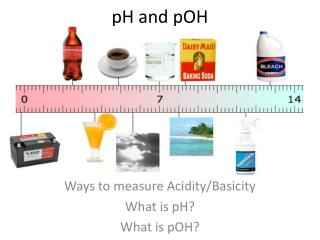DownloadDownload PresentationpH and pOH

# pH and pOH

Télécharger la présentation## pH and pOH

- - - - - - - - - - - - - - - - - - - - - - - - - - - E N D - - - - - - - - - - - - - - - - - - - - - - - - - - -
##### Presentation Transcript

1. pH and pOH Ways to measure Acidity/Basicity What is pH? What is pOH?

2. What is pH? • pH = potential Hydrogen • The pH scale measures how acidic or basic a substance is, is logarithmic • The pH scale ranges from 0 to 14. • A pH of 7 is neutral. • A pH less than 7 is acidic, greater than 7 is basic.

3. Basic Equations pH + pOH = 14 Kw = [H+] [OH-] = 1 x 10 -14

4. Calculating pH Values • pH = 14 – pOH • pH = - log [H+] Example: Calculate pH given [H+] = 1.4 x 10-5 M pH = - log [H+] pH = - log [1.4 x 10-5] pH = 4.85

5. Calculating [H+] values EXAMPLE: Find [H+] if pH = 8.5 [H+] = 10-pH [H+] = 10-8.5 [H+] = 3.2 x 10-9 M

6. Calculating pOH • pOH = 14 – pH • pOH = -log[OH-] EXAMPLE: What is the pOH of a solution that has a hydroxide ion concentration of 4.82 x 10-5M ? pOH =  - log [OH-] pOH =  - log [4.82 x 10-5] pOH = 4.32

7. YOU TRY! • Find the pH of the solution if [H+] = 1 x 10-4 M • What is the hydrogen ion concentration if pH = 7.1? • What is the pH if [OH-] = 4 x 10 -11 M? pH = 4 7.1 = -log [H+] 10 – 7.1 = [H+] = 7.9 x 10-8 M pOH = -log [OH-] pOH = -log [4 x 10 -11 ] =